Practice Questions: Parallel Operation of Thyristors

# Practice Questions: Parallel Operation of Thyristors

Test Description

## 5 Questions MCQ Test Power Electronics | Practice Questions: Parallel Operation of Thyristors

Practice Questions: Parallel Operation of Thyristors for Electrical Engineering (EE) 2023 is part of Power Electronics preparation. The Practice Questions: Parallel Operation of Thyristors questions and answers have been prepared according to the Electrical Engineering (EE) exam syllabus.The Practice Questions: Parallel Operation of Thyristors MCQs are made for Electrical Engineering (EE) 2023 Exam. Find important definitions, questions, notes, meanings, examples, exercises, MCQs and online tests for Practice Questions: Parallel Operation of Thyristors below.
Solutions of Practice Questions: Parallel Operation of Thyristors questions in English are available as part of our Power Electronics for Electrical Engineering (EE) & Practice Questions: Parallel Operation of Thyristors solutions in Hindi for Power Electronics course. Download more important topics, notes, lectures and mock test series for Electrical Engineering (EE) Exam by signing up for free. Attempt Practice Questions: Parallel Operation of Thyristors | 5 questions in 15 minutes | Mock test for Electrical Engineering (EE) preparation | Free important questions MCQ to study Power Electronics for Electrical Engineering (EE) Exam | Download free PDF with solutions
 1 Crore+ students have signed up on EduRev. Have you?
Practice Questions: Parallel Operation of Thyristors - Question 1

### SCR with a rating of 1200 V and 250 A are available to be used in a string to handle 8 kV and 1kA. The number of parallel and series units required are respectively _____ (Take derating factor is 0.2)

Detailed Solution for Practice Questions: Parallel Operation of Thyristors - Question 1

Derating factor = 1 – string efficiency*Answer can only contain numeric values
Practice Questions: Parallel Operation of Thyristors - Question 2

### The figure below shows two thyristors each rated 500 A (continuous) sharing a load current. current through thyristor T2 is 120 A the current through T1 will be nearly equal to ___ (in A)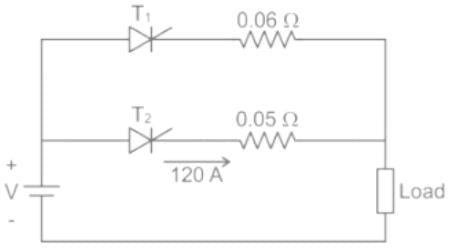Detailed Solution for Practice Questions: Parallel Operation of Thyristors - Question 2

Concept

Matched-pair SCR’s are generally available for parallel connection, but they are very expensive. With unmatched SCR’s equal current sharing is enforced by adding a low-value resistor or inductor in series with each SCR. Forced current sharing using equal-value resistors is shown in Figure 2. The basic requirement is to make current I1 close to l2, a maximum difference of 20% is accept across SCR2, the value of R can be obtained from.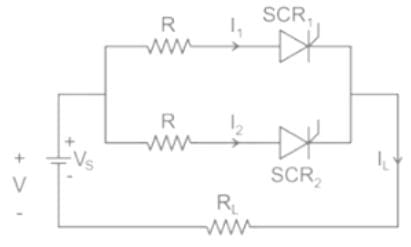I1R + V1 = I2R + V2

R = (V1 – V2)/(I2 – I1)

Calculation:

Voltage across 0.05 ohm resistor  = 120 × 0.05 = 6 V
⇒ I2 = 6/0.06 = 100 A
Current through T1 will be nearly equal to 100  A

*Answer can only contain numeric values
Practice Questions: Parallel Operation of Thyristors - Question 3

### A 300 A thyristor is to be operated in parallel with a 400 A thyristor their on state voltage drops are 1.8 V and 1 V respectively. The resistance to be connected in series with each thyristor so that the current through the combination is 700 A and each of them is fully loaded is ___________(in mΩ)

Detailed Solution for Practice Questions: Parallel Operation of Thyristors - Question 3

Concept

Matched-pair SCR’s are generally available for parallel connection, but they are very expensive. With unmatched SCR’s equal current sharing is enforced by adding a low-value resistor or inductor in series with each SCR. Forced current sharing using equal-value resistors is shown in Figure 2. The basic requirement is to make current I1 close to l2, a maximum difference of 20% is accept across SCR2, the value of R can be obtained from.I1R + V1 = I2R + V2

R = (V1 – V2)/(I2 – I1)

Calculation:

In parallel anode to cathode voltage drops are same

1.8 + 300 R = 1.0 + 400 R

⇒ 100 R = 0.8

⇒ R = 0.008 = 8 mΩ

*Answer can only contain numeric values
Practice Questions: Parallel Operation of Thyristors - Question 4

A 200 A thyristor is to be operated in parallel with a 300 A thyristor their on state voltage drops are 1.5 V and 1.2 V respectively. What is the value of resistance to be connected in series with each thyristor so that the current through the combination is 500 A and each of them is fully loaded. ____ (in Ω)

Detailed Solution for Practice Questions: Parallel Operation of Thyristors - Question 4

Concept

Matched-pair SCR’s are generally available for parallel connection, but they are very expensive. With unmatched SCR’s equal current sharing is enforced by adding a low-value resistor or inductor in series with each SCR. Forced current sharing using equal-value resistors is shown in Figure 2. The basic requirement is to make current I1 close to l2, a maximum difference of 20% is accept across SCR2, the value of R can be obtained from.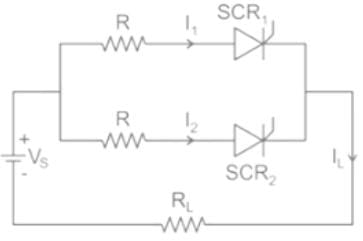I1R + V1 = I2R + V2

R = (V1 – V2)/(I2 – I1)

In parallel anode to cathode voltage drops are same

1.5 + 200 R = 1.2 + 300 R

⇒ R = 0.003 Ω

*Answer can only contain numeric values
Practice Questions: Parallel Operation of Thyristors - Question 5

It is required to operate 150 A SCR in parallel with 250 A SCR with their respective ON-State voltage drop of 1.2 V and 0.8 V. Calculate the value of resistance to be inserted in series with each SCR so that they share the total load of 400 A in proportion to their current ratings.___(in Ω)

Detailed Solution for Practice Questions: Parallel Operation of Thyristors - Question 5

Dynamic resistance of 150 A. SCR1 = 1.2/150 = 8 mΩ

Dynamic resistance of 250 A SCR 2 = 0.8/250
= 3.2 mΩ

Let RS be the resistance inserted in series with each SCR with this
Current shared by SCR1 =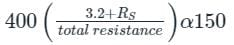Current shared by SCR2 =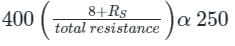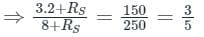⇒ 16 + 5 Rs = 3 Rs + 24
⇒ Rs = 0.004

## Power Electronics

5 videos|39 docs|63 tests
 Use Code STAYHOME200 and get INR 200 additional OFF Use Coupon Code
Information about Practice Questions: Parallel Operation of Thyristors Page
In this test you can find the Exam questions for Practice Questions: Parallel Operation of Thyristors solved & explained in the simplest way possible. Besides giving Questions and answers for Practice Questions: Parallel Operation of Thyristors, EduRev gives you an ample number of Online tests for practice

## Power Electronics

5 videos|39 docs|63 tests